# One Sample T-Test Calculator

Unknown standard deviation
or or enter summarized data (x̅, n, σ, S) below

## Enter sample data

Header: You may change groups' name to the real names.
Data: When entering data, press Enter after each value.

The tool will not count empty cells or non-numeric cells.

## Enter sample data

You may copy data from Excel, Google sheets or any tool that separate data with Tab and Line Feed.
Copy the data, one block of 2 consecutive columns includes the header, and paste below.

Copy the data,

It is okay to leave empty cells, empty cells or non numeric cells won't be counted

When entering raw data, the tool will run the Shapiro-Wilk normality test and calculate outliers, as part of the test calculation.
validation message

## Information

Target: To check if the assumed μ0 is statistically correct, based on a sample average.
You don't know the standard deviation, hence you use the sample standard deviation.

Hypotheses
H0: μ = μ0
H1: μ < > μ0
Test statisticT distribution (Student)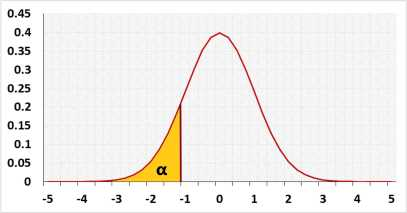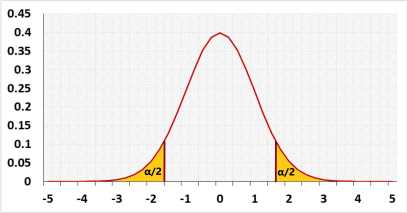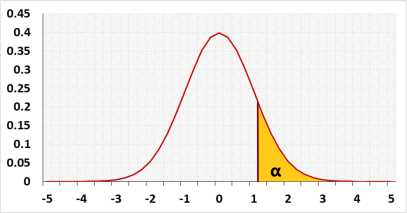## AssumptionsNormal distributionIndependent observationsThe population expected mean is known

## Required Sample Data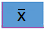Sample average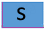Sample standard deviationSample size

## R Code

The following R code should produce the same results:

Currently, there is no direct R function for the one-sample z test.

Examples
1. Two-tailed test
A farmer calculated last year the average of the apples' weight in his apple orchard μ0 equals 17 kg, based on the entire population.
The current year he checked a small sample of apples and the sample average x̅ equals 18 kg
Has the average of the apple's weight changed this year?
The farmer calculates the sample standard deviation of the apple's weight.

2. Left-tailed test.
In the same example as above, the farmer only cares to know if the entire average is lesser this year.